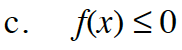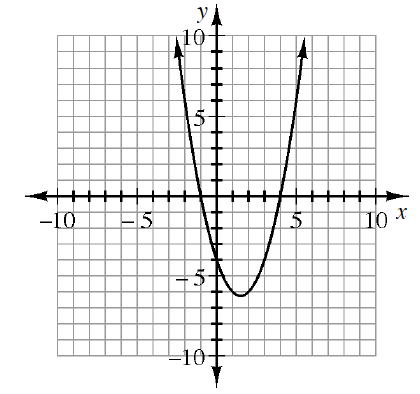### Home > INT3 > Chapter 12 > Lesson 12.2.2 > Problem12-103

12-103.
1. The graph of f(x) = x2 – 3x – 4 is shown below. Use the graph to solve: Homework Help ✎

2.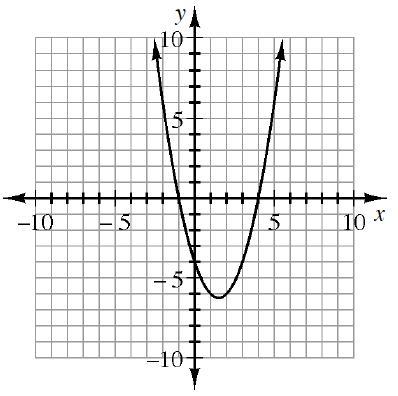1. f(x) = 0

2. f(x) ≥ 0

3. f(x) ≤ 0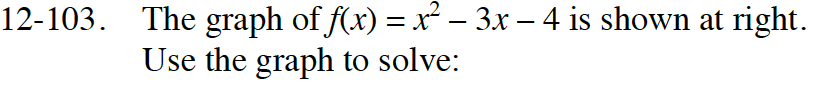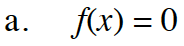Think of f(x) as y.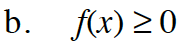x ≤ −1 or x ≥ 4